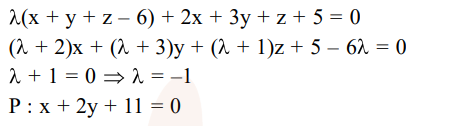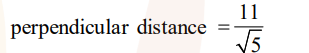# Solve this following`
Question:

Let $P$ be the plane, which contains the line of intersection of the planes, $x+y+z-6=0$ and $2 \mathrm{x}+3 \mathrm{y}+\mathrm{z}+5=0$ and it is perpendicular to the $x y$-plane. Then the distance of the point $(0,0,256)$ from $P$ is equal to :-

1. $63 \sqrt{5}$

2. $205 \sqrt{5}$

3. $17 / \sqrt{5}$

4. $11 / \sqrt{5}$

Correct Option: , 4

Solution: